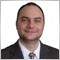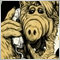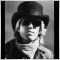# How to calculate Stop Loss pips from amount of money given437

Stop Loss calculated from money given is useful in two cases:

1. Fixing a Stop Loss to a predefined value to risk. Let's say \$80.

2. Stop Loss as percentage of the current account balance.

Here is the formula for calculating stop pips from account percentage:

```///
/// The Account Percent Stop limits the loss to a
/// predefined percent of the account balance.
/// Returns the number of pips for the StopLoss.
double AccountPercentStopPips(string symbol, double percent, double lots)
{
double balance  = AccountBalance();
double exchrate = AccountExchangeRate(symbol);
double lotsize  = MarketInfo(symbol, MODE_LOTSIZE);
double point    = MarketInfo(symbol, MODE_POINT);

double stopLossPips = percent * balance * exchrate / (lots * lotsize * point) - spread;

return (stopLossPips);
}```

There is a challenge in this simple at first task - Exchange Rate. The input parameter "Money to risk" is in the account currency but the result of the trade (profit or loss) is in the quoted currency, so there is three possibilities:

- Equal account and quoted currency: Example Account in USD and symbol EURUSD. Here the Exchange Rate = 1 because the profit is in the currency of the account.

- Account currency is the base currency: Account in USD and symbol EURUSD. Here the exchange rate is the current bid price.

- The account currency doesn't take part of the instrument being traded. Example: Account in EUR and instrument USDJPY. Here the Exchange rate has to be the current EURJPY rate.

Here is the code for implementing this formulas:

```/// Gets the Account Exchange Rate
/// AccountExchangeRate = AccountCurrency / QuotedCurrency
/// It serves to convert the profit of a deal in account currency.
/// This code has to be improved!
double AccountExchangeRate(string symbol)
{
if (StringLen(symbol) != 6)
return (1);

string accCurrency    = AccountCurrency();
string baseCurrency   = StringSubstr(symbol, 0, 3);
string quotedCurrency = StringSubstr(symbol, 3, 3);

if (accCurrency == quotedCurrency)
return (1);
else if (accCurrency == baseCurrency)
return (MarketInfo(symbol, MODE_BID));
else
{
string pair = StringConcatenate(accCurrency, quotedCurrency);
double rate = MarketInfo(pair, MODE_BID);

LastError = GetLastError();
if (LastError == 4106)
{
pair = StringConcatenate(quotedCurrency, accCurrency);
rate = MarketInfo(pair, MODE_BID);
LastError = GetLastError();
if (LastError == 0)
rate = 1 / rate;
else
rate = 1;
}
else if (LastError != 0)
rate = 1;

return (rate);
}

return (1);
}
```

This code works fine for the majority of the forex symbol but has two drawbacks:

1. It doesn't work for indexes, shares, metals..

2. Its too complicated and slow.

Mu question is if there is any MaketInfo magic for converting account currency to pips that works in general. I mean for all possible account currencies and instruments.2325

Miroslav_Popov wrote >>

This code works fine for the majority of the forex symbol but has two drawbacks:

1. It doesn't work for indexes, shares, metals..

2. Its too complicated and slow.

Mu question is if there is some MaketInfo magic for converting account currency to pips that works in general. I mean for all possible account currencies and instruments.

MarketInfo(symbol,MODE_TICKVALUE) is what u r looking for... It's the value of one tick of the quote currency in the deposit currency. So in your original function it should be something like this (I am not home so can't verify this...):

```double AccountPercentStopPips(string symbol, double percent, double lots)
{
double balance   = AccountBalance();
double tickvalue = MarketInfo(symbol, MODE_TICKVALUE);
double lotsize   = MarketInfo(symbol, MODE_LOTSIZE);

double stopLossPips = percent * balance / (lots * lotsize * tickvalue) - spread;

return (stopLossPips);
}```437

Thank you Gordon, I'll check it.

It will be perfect if it works in all the cases available.1574

Seconded.

One point - I now always implement tickvalue/ticksize as a ratio as I have (rarely) seen tickvalue change in magnitude. In this case there was also a compensatory change in ticksize.

CB2325

cloudbreaker:

Seconded.

One point - I now always implement tickvalue/ticksize as a ratio as I have (rarely) seen tickvalue change in magnitude. In this case there was also a compensatory change in ticksize.

CB

I have never myself seen changes in ticksize (and a corresponding change in tickvalue). My current projects implement client-side stoploss (some of my partners are paranoid about brokers stoploss-hunting their ass), hence no need to use tickvalue to calculate the stops (the expert just looks directly at equity).

Anyway, here's the amended function for this rare occasion of ticksize and tickvalue fluctuations:

```double AccountPercentStopPips(string symbol, double percent, double lots)
{
double balance   = AccountBalance();
double tickvalue = MarketInfo(symbol, MODE_TICKVALUE);
double lotsize   = MarketInfo(symbol, MODE_LOTSIZE);
double point     = MarketInfo(symbol, MODE_POINT);
double ticksize  = MarketInfo(symbol, MODE_TICKSIZE);

// fix for extremely rare occasion when a change in ticksize leads to a change in tickvalue
double reliable_tickvalue = tickvalue * point / ticksize;

double stopLossPips = percent * balance / (lots * lotsize * reliable_tickvalue ) - spread;

return (stopLossPips);
}
```

I have (rarely) seen tickvalue change in magnitude. In this case there was also a compensatory change in ticksize.

CB, isn't it the other way around - a change of magnitude in ticksize brings the change in tickvalue?

Anyway, I completely agree with your method, but don't u find this thread disturbing -> https://www.mql5.com/en/forum/122784 ?1574

Yes - I wasn't commenting at all on which causes which ;-) On your other point, even though you use a client side SL, you may wish to use TL and TS when calculating lot size as a % of balance to risk based on the SL you've set. CB2325

cloudbreaker:
Yes - I wasn't commenting at all on which causes which ;-) On your other point, even though you use a client side SL, you may wish to use TL and TS when calculating lot size as a % of balance to risk based on the SL you've set. CB

Agreed.

p.s. I imagine TL is supposed to be TV = tickvalue, TS = ticksize... Cause usually TL = trendline and TS = trailing stop. For a Second there I had no idea what u were talking about :-)437

CB I'm not sure I understand what you mean with this *you may wish to use TL and TS when calculating lot size as a % of balance to risk based on the SL you've set*.

The final picture must be something like this:

```double AccountPercentStopPips(string symbol, double percent, double lots)
{
double balance      = AccountBalance();
double moneyrisk    = balance * percent / 100;
double point        = MarketInfo(symbol, MODE_POINT);
double ticksize     = MarketInfo(symbol, MODE_TICKSIZE);
double tickvalue    = MarketInfo(symbol, MODE_TICKVALUE);
double tickvaluefix = tickvalue * point / ticksize; // A fix for an extremely rare occasion when a change in ticksize leads to a change in tickvalue

double stoploss = moneyrisk / (lots * tickvaluefix ) - spread;

if (stoploss < MarketInfo(symbol, MODE_STOPLEVEL))
stoploss = MarketInfo(symbol, MODE_STOPLEVEL); // This may rise the risk over the requested

stoploss = NormalizeDouble(stoploss, 0);

return (stoploss);
}
```20384

Set the stop loss based on the market. Set the lot size based on risk. Tickvalue changes if the account currency isn't in the symbol.
```//+------------------------------------------------------------------+
//| Lot size computation.                                            |
//+------------------------------------------------------------------+
double  LotSize(double SL_points, bool modifying = false) {
/* This function computes the lot size for a trade.
* Explicit inputs are SL relative to bid/ask (E.G. SL=30*points,) and if
* returned lot size will be used in a new order or be used to modify an
* pending order (trade count-1.) Implicit inputs are the MM mode, the MM
* multiplier and currently filled or pending trade count (used to reduce
* available balance.) Mode, multiplier, adjusted account balance determined
* the maximum dollar risk allowed. StopLoss determines the maximum dollar
* risk possible per lot. Lots=maxRisk/maxRiskPerLot
**************************************************************************/
double  ab  = AccountBalance();
switch(MMMode_F0M1G2) {
case MMMODE_FIXED:
atRisk  = MMMultplier;
break;
case MMMODE_MODERATE:
// See https://www.mql5.com/en/articles/1526 Fallacies, Part 1: Money
// Management is Secondary and Not Very Important.
atRisk  = MathSqrt(MMMultplier * ab)/ab;    // % used/trade ~const.
atRisk  = MathSqrt(MMMultplier * ab
* MathPow( 1 - atRisk, OrdersTotal()-modifying ));
break;
case MMMODE_GEOMETRICAL:
atRisk  = MMMultplier * ab
* MathPow(1 - MMMultplier, OrdersTotal()-modifying);
break;
}
double  maxLossPerLot   = SL_points/Point
* MarketInfo( Symbol(), MODE_TICKVALUE );
// IBFX demo/mini       EURUSD TICKVALUE=0.1 MAXLOT=50 LOTSIZE=10,000
// In tester I had a sale: open=1.35883 close=1.35736 (0.00147)
// gain\$=97.32/6.62 lots/147 points=\$0.10/point or \$1.00/pip.
// IBFX demo/standard   EURUSD TICKVALUE=1.0 MAXLOT=50 LOTSIZE=100,000
//                                  \$1.00/point or \$10.00/pip.
double  LotStep = MarketInfo( Symbol(), MODE_LOTSTEP ),
// atRisk / maxLossPerLot = number of lots wanted. Must be rounded/truncated
// to nearest lotStep size.
//
// However, the broker doesn't care about the atRisk/account balance. They
// care about margin. margin used=lots used*marginPerLot and that must be
// less than free margin available.
marginFree   = AccountFreeMargin(),                     // Free Margin
marginPerLot = MarketInfo( Symbol(), MODE_MARGINREQUIRED ),
// So I use, the lesser of either.

// I don't use MODE_MAXLOT as OpenNow handles that.
size =  MathFloor(  MathMin ( marginFree / marginPerLot
, atRisk     / maxLossPerLot )
/ LotStep )*LotStep;        // truncate
if (size < MarketInfo( Symbol(), MODE_MINLOT )) {
// Multiple orders -> no free margin -> LotSize(SL=4.1)=0
// [risk=9.48USD/40.80, margin=10.62/1334.48, MMM=1x1, coat=0]
//       0.23                  0.007
Print(
"LotSize(SL=", DoubleToStr(SL_points/pips2dbl, Digits.pips), ")=",
size, " [risk=",    atRisk, AccountCurrency(),  "/", maxLossPerLot,
", margin=",    marginFree,             "/", marginPerLot,
", MMM=",       MMMode_F0M1G2,          "x", MMMultplier,
", coat=",      OrdersTotal(),  // Count Of active trades
"]" );
return(0.0);    // Risk limit.
}
atRisk  = size * maxLossPerLot; // Export for Comment()
return(size);
}   // LotSize
```110

gordon:

I have never myself seen changes in ticksize (and a corresponding change in tickvalue). My current projects implement client-side stoploss (some of my partners are paranoid about brokers stoploss-hunting their ass), hence no need to use tickvalue to calculate the stops (the expert just looks directly at equity).

Anyway, here's the amended function for this rare occasion of ticksize and tickvalue fluctuations:

CB, isn't it the other way around - a change of magnitude in ticksize brings the change in tickvalue?

Anyway, I completely agree with your method, but don't u find this thread disturbing -> https://www.mql5.com/en/forum/122784 ?

```  double Lots(string symbol, double percent, int SL)
{
double balance   = AccountFreeMargin();// more conservative... may use equity or balance
double tickvalue = MarketInfo(symbol, MODE_TICKVALUE);
double point     = MarketInfo(symbol, MODE_POINT);
double ticksize  = MarketInfo(symbol, MODE_TICKSIZE);

double CBtickvalue = tickvalue * point / ticksize;

double lots  = percent * balance /  ( SL + spread ) * CBtickvalue  ;

lots = NormalizeDouble(lots,1);

return (lots);

}```20384

cloudbreaker:
One point - I now always implement tickvalue/ticksize as a ratio as I have (rarely) seen tickvalue change in magnitude. In this case there was also a compensatory change in ticksize.

Must be implemented as a ratio. Here's an example where ticksize=0.5

Also pending order prices must be a multiple of ticksize.

Also do NOT normalizeDouble(lots,1) It MUST be a multiple of lotStep.